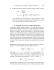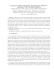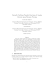# HOMEWORK PROBLEMS 8 PHYS 516, Fall 2014

## Transcription

HOMEWORK PROBLEMS 8 PHYS 516, Fall 2014
```HOMEWORK PROBLEMS 8
PHYS 516, Fall 2014
Special Functions and Partial Differential Equations
Due date: Monday, 11/24/14 — 3.00pm (i.e. in class)
1. (a) Arfken & Weber Problems 11.7.8 and 12.4.10. You may need to read
the start of Section 11.7 to remind yourself about the definition of spherical
Bessel functions.
(b) Then use your result from 12.4.10 to prove the integral identity
s
In+1/2 (z) =
z
2π
Z 1
−1
ezx Pn (x) dx
(1)
for the modified Bessel function.
[24 points credit]
2. Prove Laplace’s useful integral representation of the Legendre function of
the first kind Pn (z)
Z
in
√
1 πh
Pn (z) =
z + z 2 − 1 cos φ dφ , |z| > 1 ,
(2)
π 0
by showing that it satisfies the generating function relation
√
∞
X
1
=
tn Pn (z) ,
2
1 − 2tz + t
n=0
|t| < 1 .
(3)
Hint: you will need to perform a contour integration, the form of which you
have seen before.
[15 points credit]
1
3. Arfken & Weber Problems 13.1.12 and 13.1.18. For 13.1.18, use instead
the function f (x) = x6 to determine the coefficients an , and then check
your results using Table 13.1.
[25 points credit]
4. Arfken & Weber Problems 9.3.6 & 9.3.7. Then identify appropriate eigenvalues (or quantum numbers) to express the separation constant, so that the
general solution can be written as an infinite series of well-known special
functions.
If the boundary condition for the problem is that the temperature is exactly
zero at the outer radius R of the cylinder, discuss how this constrains the
form of the solution.
[16 points credit]
5. (a) Using the technique of separation of variables, show that the solution
to the three-dimensional diffusion equation for temperature T , in the special
case of cylindrical symmetry about an axis r = 0 , assumes the form (for µ
to be specified)
T (r, t) = rµ
Z ∞
0
dk f (k) J0 (k r) e−k
2 κt
,
(4)
where f (k) is an arbitrary function of the dimensionless integration variable
k , κ is the diffusion coefficient, and J0 (z) is the ordinary Bessel function of
order zero.
(b) Using a familiar integral representation of the ordinary Bessel functions,
prove the Laplace transform identity
Z ∞
0
1
e−αk J0 (k r) dk = √ 2
α + r2
.
Hint: Part of the analysis of Problem 2 should come in handy.
2
(5)
(c) Consider cases where f (k) ∝ k . Use appropriate derivatives of the
Laplace transform identity to demonstrate that such cases are appropriate
for the diffusion problem where the heat (i.e. temperature) is initially (i.e.
at t = 0 ) infinitely concentrated at r = 0 , and zero elsewhere. Can you
determine a simple analytic form for T (r, t) for such scenarios?
[20 points credit]
3
```

### AMATH 351 Autumn 2014 Homework 5 Due: Wednesday, November 5, 2014, 10:30AM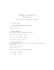### This redemp on is for the Weber “Grills and Thrills” Promo on. Send in t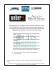### MAT 132 - Calculus II,Practice Problems for Midterm 2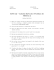### Homework 5A: Green`s functions Math 456/556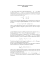### Diffusion Lab Questions AP Biology Name________________________ 1. What is Kinetic Energy?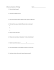### HW4Solutions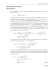### Laplace Transforms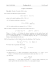### Sample Problems = +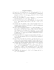### Calculus I Final, Sample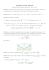### Horseware® Ireland Rides with Team Weber for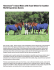### Math 220 Sample Exam 4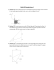### Chester Weber and Live Oak International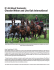### Document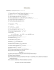### Optical vortices with large orbital momentum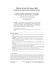### Opinion - Florida State University College of Law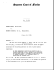### Ablowitz and Fokas, Section 4.6